• 利用指针访问多维字符串数组的问题，是一个有趣且重要的知识点，其实并不复杂只需要对指向指针的指针进行拆解分析即可，下面进入正文 首先我们定义一个多维字符串数组，然后利用4种方式进行输出 #include <...
利用指针访问多维字符串数组的问题，是一个有趣且重要的知识点，其实并不复杂只需要对指向指针的指针进行拆解分析即可，下面进入正文
首先我们定义一个多维字符串数组，然后利用4种方式进行输出
#include <iostream>
using namespace std;
int main() {
char t[] = {"abcd","efgh","ijkl","mnop","qrst","uvwx"};
cout<<t<<" "
<<*(t+2)<<" "
<<*(*(t+2)+2)<<" "
<<(*(*(t+1)+2)+2)<<endl;
return 0;
}
/*
输出结果
w w w wx
*/

首先字符串数组会自动被分成三维数组 也可以简单想成两行三列的数组，只是每个元素都是一个字符串也就是字符型数组。接下来我们可以看到第4种的输出与其他的不同，接下来我们进行分析首先我们要明确 数组名 是数组第一个元素的地址 利用如下代码进行理解
#include <iostream>
using namespace std;
int main() {
int a[]={1,2,3,4,5,6};
cout<<a<<" "<<*a<<" "<<*(a+3)<<endl;
return 0;
}
/*
输出结果
0x7ffee2d57830 1 4
*/

对四个输出分别进行分析

最基本的多维数组通过数组下标进行访问，访问的是第一维下标为1 第二维下标为2 第三维 字符串中下标为2的第三个字母’w’。

首先我们要知道t中的内容为"uvwx"这个字符串，字符串也是一个数组，也就意味着t是一个指向"uvwx"这个字符串首位的指针。t+2意味着将这个指针向后移2位，指向’w’ 。*(t+2)获取这个指针当中的内容便取出w。可以使用如下代码进行理解
#include <iostream>
using namespace std;
int main() {
char t2={'a','b','c','d','e','\0'};
cout<<t2<<' '<<*t2<<' '<<*(t2+2)<<endl;
return 0;
}
/*
输出结果
abcde a c
*/


首先t同样作为一个数组是一个包含三个指向字符串的指针。而将t理解为数组名，它指向的地址空间中存放着一个指向"mnop"字符串的指针，t+2 将指针向后移2位即为指向"uvwx"字符串的指针。*(t+2)即为取得指向“uvwx”字符串的指针所指向地址空间中的内容，即为“uvwx”这个字符串。而 *(*(t+2)+2)与第二个输出相类似，进行相同理解即可，所以输出w。

首先t可以近似理解成是一个存放着两个指针的数组，*(t+1)与t意思相同，中间部分理解与第三输出类似，故不再进行赘述。而重点在最后部分，*(*(t+1)+2)是"uvwx"这个字符串，而其中要关键要注意的便是(“uvwx”+2)与*(“uvwx”+2)的区别。未加星号的意味着仅将指针向后移两位，输出时会按照字符串进行输出到’\0’停止，而加星号则是获取指针后移2后那一位地址空间中的内容。可以利用如下代码进行理解。
#include <iostream>
using namespace std;
int main() {
cout<<"abcde"+2<<endl;
char t2={'a','b','c','d','e','\0'};
cout<<t2+2<<' '<<*(t2+2)<<endl;
return 0;
}
/*
输出结果
cde
cde c
*/

以上均属于个人理解，如果有问题欢迎大家与我进行讨论。


展开全文指针
• 多维数组 iArray = zeros(2,3); %定义2行3列的数组，未赋值的默认为0. iArray(1,:) = [1 2 3]; %给第一行的数组赋值 iArray(2,3) = 4; %给第二行第三个元素赋值 disp(iArray); 结果 cell元包数组 strArray = ...
多维数组
iArray = zeros(2,3);     %定义2行3列的数组，未赋值的默认为0.

iArray(1,:) = [1 2 3];   %给第一行的数组赋值
iArray(2,3) = 4;         %给第二行第三个元素赋值

disp(iArray);

结果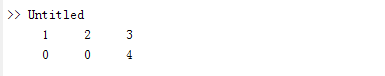cell元包数组
strArray = cell(2,3);   %定义一个cell元胞数组,这里可以存各种类型。

strArray(1,:) = {'aa','bb','cc'};
strArray(2,3) = {'zz'};

disp(strArray);

注意这里使用的是“{}”
结果：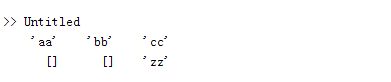结构体
structArray(1).name = '张三';
structArray(1).age = 12;
structArray(1).other = strArray;

structArray(2).name = '李四';
structArray(2).age = 13;
structArray(2).other = strArray;

for i=1:length(structArray)
disp(structArray(i).name);
disp(structArray(i).age);
disp(structArray(i).other);

end

注意，下标是从1开始的。
结果：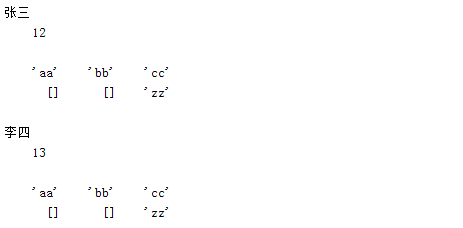展开全文matlab
• <p>In my project I need to read a value as a global variable so I am using maps (global variable) <pre><code>var url = make(map[string]string) </code></pre> <p>and I occasionally get into error ...arrays string dictionary
• 仅作为操作记录，大佬请跳过。 文章目录直接上代码背景参考 ...博主想把数组： “阴虚质”, “气虚质”, “阳虚质” 变成 “阴虚质,气虚质,阳虚质” 通过上面一行代码即可完成。 参考 传送门 ...
仅作为操作记录，大佬请跳过。
文章目录直接上代码背景参考
直接上代码
string textsavejoin = string.Join(" ", textsave);

其中textsave是string[]型数组：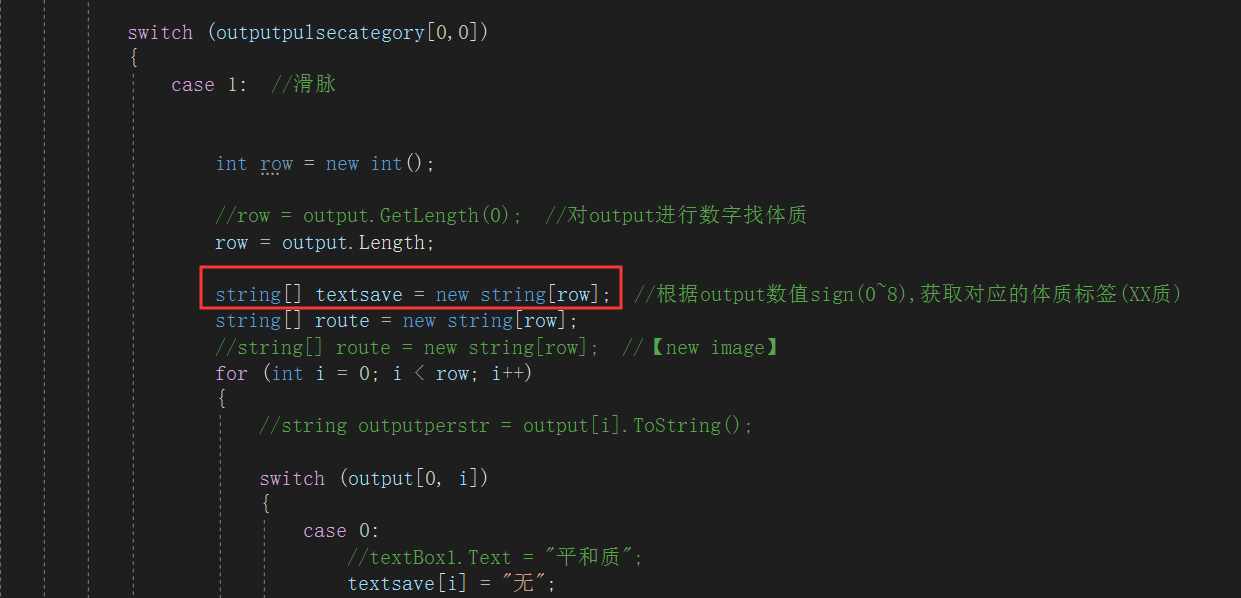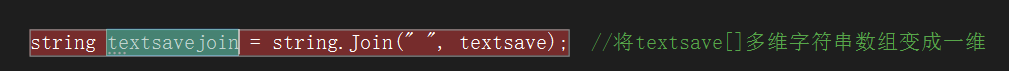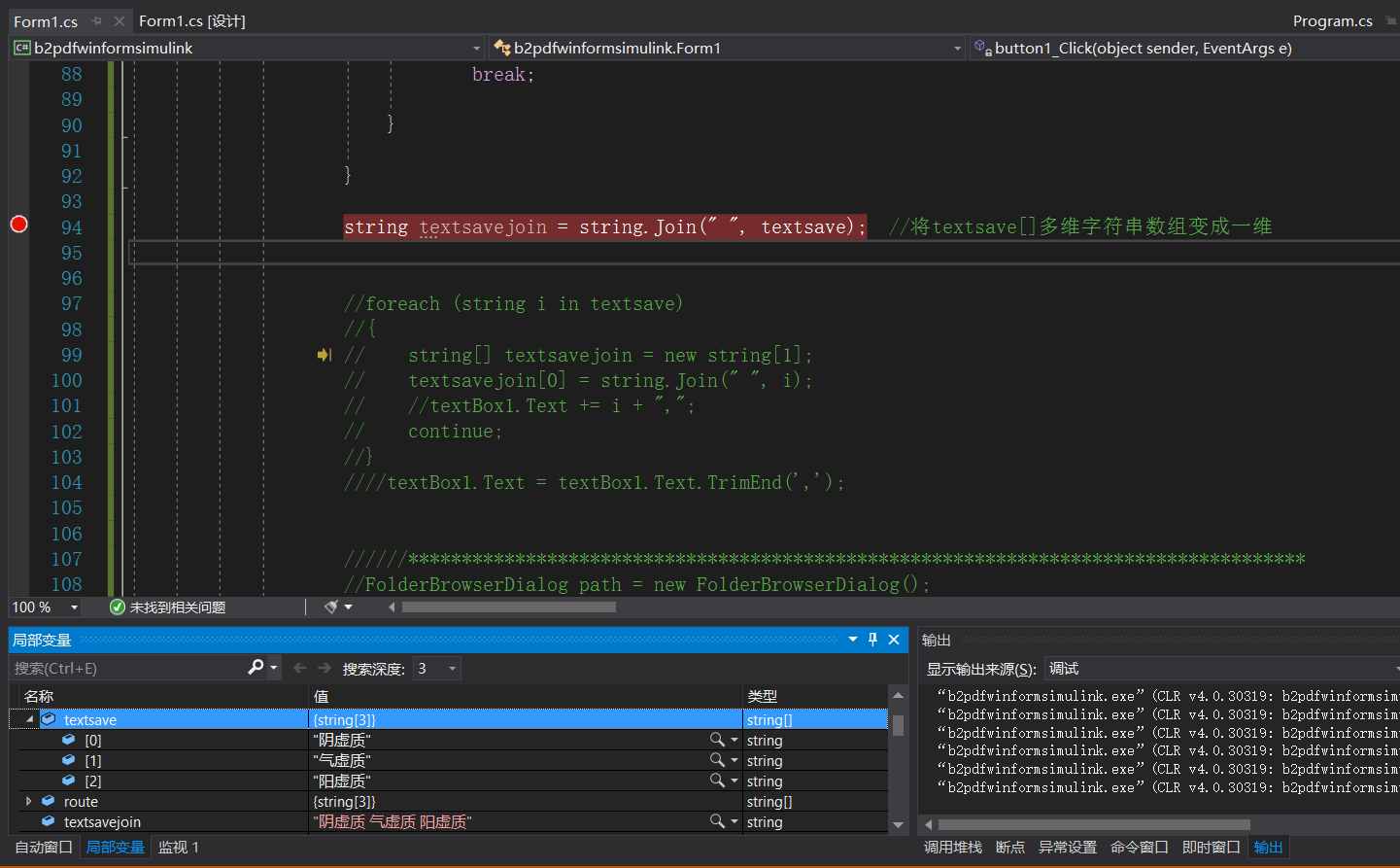背景
博主想把数组：
“阴虚质”, “气虚质”, “阳虚质”
变成
“阴虚质,气虚质,阳虚质”
通过上面一行代码即可完成。
参考
传送门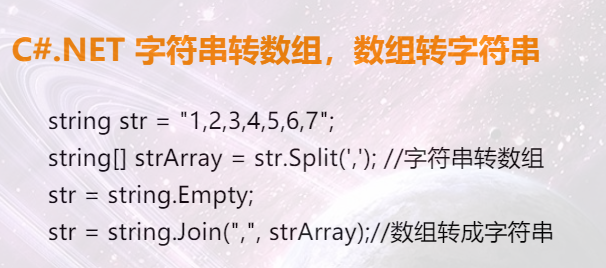展开全文• 二维数组，多维数组，字符串数组的简介

//二维数组，多维数组，字符串数组；
//二维数组：
//       二维数组的概念：数组名后面有两个下标的数组被称为二维数组
//       定义：类型说明符数组名[常量表达式1][常量表达式2]={值1，值2，....}
//       其中常量表达式1代表的是一维的长度，常量表达式2代表的是二维的长度
//二维数组定义时，如果元素未一一列出，未赋值的元素自动初始化为0

3）二维数组
int a={{1},{2},{3}};
for (int i=0; i<3; i++) {
for (int j=0; j<3; j++) {
printf(" a[%d][%d]=%d  ", i, j, a[i][j]);
}
printf("\n");
}

int array={0,1,2,3,4,5,6,7,8,9,10,11,12,13,14};
//
//                printf("array=%d\n",array);
//                printf("array=%d\n",array);
//                printf("array=%d\n",array);
//                printf("array=%d\n",array);
//                printf("array=%d\n",array);
//
//                printf("array=%d\n",array);
//                printf("array=%d\n",array);
//                printf("array=%d\n",array);
//                printf("array=%d\n",array);
//                printf("array=%d\n",array);
//
//                printf("array=%d\n",array);
//                printf("array=%d\n",array);
//                printf("array=%d\n",array);
//                printf("array=%d\n",array);
//                printf("array=%d\n",array);
//
//                printf("array=%d\n",array);
//
array==array
//
用for循环输出
//                int array={0};
//                for (int i=0; i<3; i++) {
//                    for (int j=0; j<5; j++) {
//                    array[i][j]=arc4random()%(30-10+1)+2;
//                        printf("array[%d][%d]=%d\n",i,j,array[i][j]);
//                    }
//                }
//
交换一个二维数组的行和列
//
//                int array={1,2,3,4,5,6,7,8,9,10,11,12,13,14,15};
//                int array1={0};
//
//                for (int i=0; i<3; i++) {
//                    for (int j=0; j<5; j++) {
//
//                        printf("array[%d][%d]=%d\n",i,j,array[i][j]);
//                        array1[j][i]=array[i][j];
//                    }
//                }
//
//                for (int i=0; i<5; i++) {
//                    for (int j=0; j<3; j++) {
//                       printf("array1[%d][%d]=%d\n",i,j,array1     [i][j]);
//                    }
//                            }

有一个三行四列的二维数组，找出最大元素，并输出所在的行和列
int max=0,k=0,l=0,g=0;
int arr={0};
for (int i=0;i<3;i++) {
for (int j=0; j<4; j++) {
arr[i][j]=arc4random()%(30-10+1)+10;
printf("arr[%d][%d]=%d\n",i,j,arr[i][j]);

if (max<arr[i][j]) {

k=arr[i][j];
max=k;
arr[i][j]=k;

l=i;
g=j;
}
}
//        若要求每一行的最大数值和行列的话，就加上这句：printf("max=%d,l=%d,g=%d\n",max,l,g);
}
printf("max=%d,l=%d,g=%d\n",max,l,g);

//多维数组：数组下标在两个以上。多维数组元素个数等于各个维数相乘的积（如int arr={0}共有24个元素）
//多维数组的遍历，几维数组就需要几层for循环0.
//多维数组元素访问：数组名[下标][下标][下标][下标]....

/**
*      回顾 ： 字符数组：用于存放字符变量的数组，字符数组也是一维数组，与与普通字符定义方式相似,只是类型是char
char 数组名[常量表达式1]={值1}{值2};
char array={'i', 'P', 'h', 'o', 'n', 'e'};
for (int i=0; i<10; i++) {
printf("array[%d]=%c\n",i,array[i]);
}

char array1="iphone6p";
for (int i=0; i<10; i++) {
printf("aaray1[%d]=%c\n",i,array1[i]);
}
有'\0'结束标识的字符数组也称为字符串；字符数组长度要比字符长度大一，用来存放'\0'标识
strlen()  计算字符串长度

字符串比较
注意：字符串比较函数是根据对应下标的字符的ASCII码值作比较（减法），如果差值为零，说明两个字符相等，接着比较下一位ASCII码值，直到遇到'\0'，如果差值不为零则返回该差值，表示两个字符是不同的
int  strcmp(<#const char *#> s1, <#const char *#> s2);
比较两个字符串的大小（不忽略大小写），返回值很有学问：如果s1小于s2返回一个小于0的数，如果s1大于s2返回一个大于0的数，如果相等则返回0。返回值是两个字符串中第一个不相等的字符ascii码的差值
查找字符串strstr(const char *dst, <#const char *#>src);
字符串复制strcpy(<#char *#>dst, const char *csc)；
字符串拼接char*strcpy(<#char *#>s1, <#const char *#>s2);
*/        //
//    //字符串赋值strcpy
//        char newstr[]={'\0'};
//        strcpy(newstr *, str);
//        printf("newstr=%s\n",newstr);
//
//        //字符串拼接
//        strcat(str,str1);
//        printf("str1=%s\n",str1);

字符串数组；用来存放字符串（以'\0'结尾的数组被称为字符串）的数组；
//字符串数组定义 char数组名[常量表达式1][常量表达式2] = {值1，值2，值3，.....}
char string={"iphone", "android" "windows"};
char string1={{'i','P','h','o','n','e', '7'},{'A', 'n', 'd', 'r', 'o', 'i', 'd'},{'w', 'i', 'n','8'}};
注意事项：字符串数组实际是字符型的二维数组
每个字符长度不超过二维长度减一（字符串需要存放'\0'）
访问字符串，数组名[第一维下标]
访问字符，数组名[第一维下标][第二维下标]
sizeof和strlen区别：strlen指字符的长度，sizeof指文件或者数据占的内存(字节)

练习2创建一个字符串数组（内容是周围人的姓名），输出最长字符串的长度
//       求字符串长度的结果数据类型是unsigned long（无符号长整形）
//        无论是赋值还是作比较，都需要双方类型一致
//       访问字符串，数组名[第一维下标]
//        char arr={"zhangpengfei", "qiaozipeng", "lizhipeng", "guoyaru", "lishuming"};
//        unsigned long max=0, k=0;
//        for (int i=0; i<5; i++) {
//            if(max<strlen(arr[i])){
//                max=strlen(arr[i]);
k=i;
//            }
//        }
//        printf("%s,max=%lu\n", arr[k],max);
//    %c是按字符格式输入和输出 //  %s是按字符串格式输入和输出
//

输入年月日，判断是一年中的第几天
int dayOfYear(int year,int month,int day){

int sum=0;

int  rundays[]={31,29,31,30,31,30,31,31,30,31,30,31};

int  days[]={31,28,31,30,31,30,31,31,30,31,30,31};

if (year%4==0 || (year%400==0 && (year%100!=0)))
{

for (int i=0; i<month-1; i++)
{
sum +=rundays[i];
}

}
else {

for (int i=0; i<month-1; i++)
{
sum +=days[i];
}
}
sum += day;

return sum;
}


展开全文• 字符数组又称字符串 字符串数组是二维数组 char a[]={'i', 'p', 'h', 'o', 'n', 'e'}; char c="iphone"; char c={"hello", "panny", "welcome", "good"};该数组包含三个字符串。 字符串数租元素...
• #pragma mark ----------二维数组----... 二维数组的本质:将数组作为数组元素的数组,称为数组数组 2 . 类如:管理一个班4个学生的年龄,将一维数组作为数组元素,存储到另一个数组中 int age = {12,13,14,15}; 12,...
• ⼆二维数组、字符串数组多维数组 转载于:https://www.cnblogs.com/jiangdaohong/p/4248219.html
• // 二维数组、字符串数组多维数组 // 讲师：小辉 // 笔者：王学文 // Created by lanouhn on 15/1/19. // Copyright (c) 2015年 lanouhn. All rights reserved. // #import <Foundation/...
• 字符串数组元素访问:  1、访问某个字符串:数组名[第⼀一维下标]  2、访问某个字符 :数组名[第⼀一维下标][第⼆二维下标] 3、多维数组占⽤用内存 = 元素个数 乘以 单个元素所占空间 创建⼀一个字符串数组(内容...
• ​ 先获取字符串数组中的最小长度的字符串的长度为 min（只存储长度为最小字符串长度的字符，节省空间），将字符串数组转换成多维字符数组，维度与字符串个数一致，字符数组的每一行代表一个字符串。 ​ 然后从字符...算法 java
• // C5_二维数组-字符串数组 // // Created by 何恩营 on 14-10-21. // Copyright (c) 2014年 lanou3g.com 蓝鸥科技. All rights reserved. // #import int main(int argc, con二维数组 c
• 如果“s”是字符数组，则可以根据分隔符（可以是单个元素或多个元素（例如 CRLF））将其分成一维字符串元胞数组。如果“s”是多维的，则行或列的“s”可以进一步划分为单元格s。如果“s”是一个单元格串数组，那么...matlab
• // C11 二维数组、字符串数组多维数组 // // Created by xalo on 15/10/21. // Copyright (c) 2015年 Darling.com. All rights reserved. // #import int main(int argc, const ...iOS ios开发 c语言
• 其实遇到的是 多维数组 然后根据循环数组里面的某一个数据进行判断 然后在渲染 最简单的方式 : 利用 var arrey1=[] arrey.forEach(item=>){ if(item==1){ //这里面写根据数据进行判断的条件 arrey1=item.shuzu...vue.js css javascript 前端 html
• 1.int a={0};数组元素有5个。 2.er'wei'shu
• // 1.(**)有一个 3 行 4 列的二维数组,要求编程找出最大的元素,并输出所 在的行和列。// int max = 0, array = {0}; // for (int i = 0; i ; i++) { // for (int j = 0; j ; j++) { // array[i][j] = arc4...c ios
• C++ 支持数组数据结构，它可以存储一个固定大小的相同类型元素的顺序集合。数组是用来存储一系列数据，但它往往被认为是一系列相同类型的变量。 数组的声明并不是声明一个个单独的变量，比如 number0、number1、......
• //打印字符串数组中全部字符串 // long maxLength = 0; // for (int i = 0; i; i++) { // printf("%s ",str[i]); // printf("%ld",strlen(str[i]));//求长度 // printf("%s",strcpy(str[i], "zhangsan"));//赋值 //...
• <p>This is the array: <pre><code>Array (  => Array ... [product_details] => {"5f93f983524def3dca464469d2cf9f3e":{"id":"110","qty":1,"option":"{\"color\":{\"title\":\"Color\",\"value\":null}...arrays json php
• 一、二维数组 1.二维数组定义： 注：常量表达式1->第一维度 常量表达式2->第二维度 可以没有第一维度的长度，但一定要写第二维度长度 若不指定第一维度，就一定要加初值 2.使用二位数组中的元素，数组...二维数组...